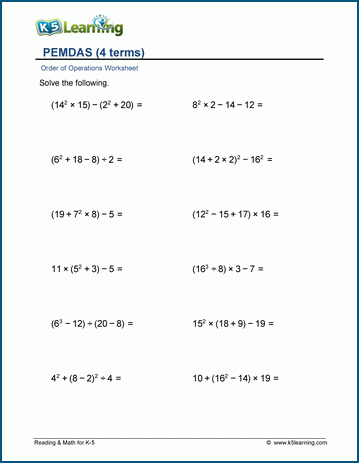# Order of operations worksheets

## Including PEMDAS

These worksheets focus on order of operations. First parenthesis are introduced to addition and subtraction equations. Then multiplication / division are added. Finally exponents are included.

## Add & subtract with parenthesis

Add / subtract 3-4 terms with parenthesis 19 - (15 - 4) =
Add / subtract 5+ terms with parenthesis 12 - (8 - 5) + 7 - 3 =

## 4 operations with parenthesis

Order of operations - 3 terms 18 x (13 - 9) =
Order of operations - 4 terms (19 - 7) x (36 ÷ 3) =
Order of operations - 5 terms 4 x (8 ÷ 2 + 5) x 3 =

## 4 operations, exponents & parenthesis

PEMDAS - 3 terms 10 + (62 - 12) =
PEMDAS - 4 terms (11 + 7) ÷ 9 x 43 =
PEMDAS - 5 terms (8+33) x (9 - 4)2÷ 5 =Sample order of operations worksheet

What is K5?

K5 Learning offers free worksheets, flashcards and inexpensive workbooks for kids in kindergarten to grade 5. Become a member to access additional content and skip ads.